# GRE Subject Test: Math : Statistics

## Example Questions

### Example Question #521 : Gre Subject Test: Math

What is the mean of: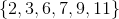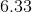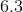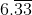Explanation:

Step 1: Find the sum of the numbers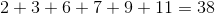Step 2: Count how numbers there are:

There arenumbers..

Step 3: Divide Sum by how many numbers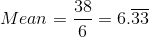### Example Question #21 : Statistics

What is the mean of: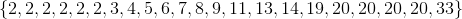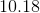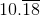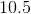Explanation:

Step 1: Find the sum of all the numbers: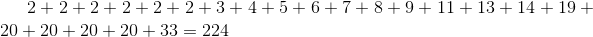Step 2: Count how many numbers there are:

There are 22 numbers.

Step 3: Divide the sum by how many numbers there are: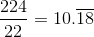The mean is### Example Question #21 : Statistics

What is the mean of: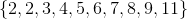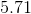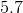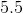Explanation:

Step 1: Take the sum of all numbers in the set...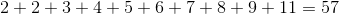Step 2: Count how many elements are in the set...

There arenumbers

Step 3: To find the mean, divide the sum by the number of elements..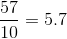The mean is### Example Question #527 : Gre Subject Test: Math

In the set,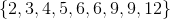, is the mean bigger than the median?

Cannot Tell

None

Yes

No

Yes

Explanation:

Step 1: Calculate the Mean..

Take the sum of all the numbers in the set...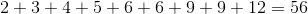Divide the sum by how many numbers there are...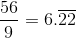=Mean
Step 2: Calculate the Median:
The median of this set is the number in the middle.

Count how many numbers are in the set...
There are...

So, the median is theth number in the set.

Theth number is.

The mean is bigger than the median, so the answer is true.

### Example Question #528 : Gre Subject Test: Math

Tommy got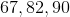on the first three tests. What must he score on the fourth test so that the average is 81?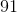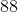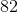Explanation:

Step 1: Find the total sum of the tests. To do this, we multiply the average by the number of tests:

Total=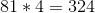Step 2: To find the missing term, we subtract each of the three test scores that are provided...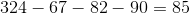.

Tommy needs anon his fourth test to get that average...

### Example Question #21 : Probability & Statistics

What is the mean of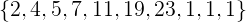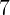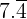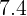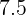Explanation:

Step 1: Find the sum of the numbers in the set...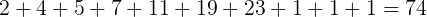Step 2: Count how many numbers are in the set..

There are 10 numbers...

Step 3: Divide the total sum by how many numbers...

So,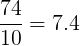### Example Question #22 : Statistics

What is the mean of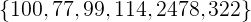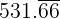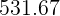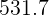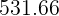Explanation:

Step 1: Find the sum of all the terms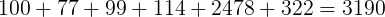Step 2: Count how many numbers are in the set

There are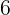numbers.

Step 3: Divide the sum (Step 1) by how many numbers in the set (Step 2)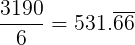### Example Question #31 : Other Topics

What is the mean of: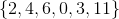?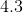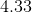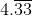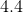Explanation:

Find the sum of all the numbers in the set: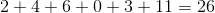Count how many numbers are in the set...

There are six numbers...

Divide the sum of the set by how many numbers to find the mean...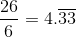### Example Question #22 : Statistics

What is the mean of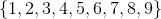?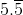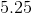NoneExplanation:

Find the sum of all the numbers in the set: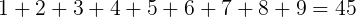Count how many numbers are in the set...

There are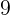numbers..

To find the mean, divide the sum by how many numbers...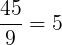### Example Question #31 : Probability & Statistics

What is the mean of: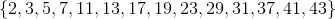?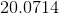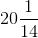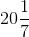Explanation:

Step 1: To find the mean, we need to first find the sum of the numbers in the set.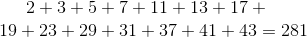Step 2: Count how many numbers are in the set...

There arenumbers in the set.

Step 3: To find the mean, divide the sum of the numbers in the set by how many numbers there are in the set...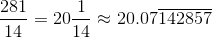### All GRE Subject Test: Math Resources